# Samacheer Kalvi 10th Maths Book Solutions Chapter 4 | Geometry Answers For Tamil Nadu Board

Samacheer Kalvi 10th Maths Book Solutions Chapter 4 – Geometry is available here. The Samacheer Kalvi 10th Maths book answers of Chapter 4, available at BYJU’S, contain step by step explanations designed by our Mathematics experts. All these important questions are based on the new pattern prescribed by the Tamil Nadu board. Students can also get the solutions of other chapters on Samacheer Kalvi 10th Maths solutions. Preparing for the board exams becomes more easy with the help of these Samacheer Kalvi Class 10 Maths Textbook Chapter 4 Questions and Solutions.

Chapter 4 of the Samacheer Kalvi 10th Maths guide will help the students to solve problems related to similarity, similar triangles, congruence and similarity of triangles, criteria of similarity, construction of similar triangles, Thales Theorem and Angle Bisector Theorem, construction of triangle, Pythagoras Theorem, Circles and Tangents.

### Samacheer Kalvi 10th Maths Chapter 4: Geometry Book Exercise 4.1 Questions and Solutions

Question 1: Check whether the triangles are similar and find the value of x.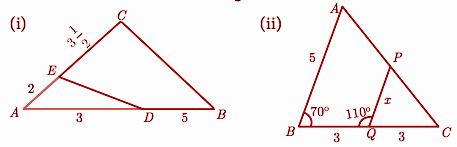Solution:

(i) Consider the △AED and △ACB

The two triangles are said to be similar if the ratio of the corresponding sides is equal.

AE / AC = AD / AB

2 / (7 / 2) = 3 / 5

4 / 7 = 3 / 5

(ii) In △PQC,

∠PQC = 180 – 110

∠PQC = 70

Consider the △ABC and △PQC.

∠ABC = ∠PQC

∠ACB = ∠PCQ

By using AA criterion, the above triangles are similar.

Hence, the ratio of their corresponding sides will be equal.

AB / PQ = BC / QC

5 / x = (3+3) / 3

5 / x = 6 / 3

5 / x = 2

x = 5 / 2 = 2.5

Question 2: A girl looks at the reflection of the top of the lamp post on the mirror which is 6.6 m away from the foot of the lamp post. The girl whose height is 1.25 m is standing 2.5 m away from the mirror. Assuming the mirror is placed on the ground facing the sky and the girl, mirror and the lamp post are in the same line, find the height of the lamp post.

Solution: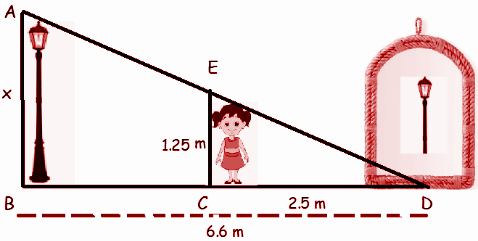In △ABD and △CED

∠ABD = ∠ECD

By AA the above triangles are similar,

AB / EC = BD / CD

x / 1.25 = 6.6 / 2.5

x = 6.6(1.25) / 2.5

x = 3.3 m

The height of the lamp post is 3.3 m.

Question 3: In the adjacent figure, triangle ABC is right-angled at C and DE ⊥ AB. Prove that ΔABC ∼ ΔADE and hence find the lengths of AE and DE.

Solution: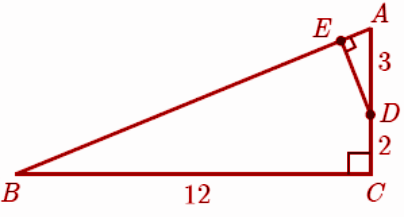In △ABC,

AB2 = BC2 + AC2

AB2 = 122 + 52

AB2 = 144 + 25 = 169

AB = 13

∠BCA = ∠AED

∠CAB = ∠DAE

So, △ABC and △EAD are similar.

BC / EA = CA / ED = AB / AD

12 / EA = (2+3) / ED = 13 / 3

12 / EA = 5 / ED = 13 / 3

12 / EA = 13 / 3

EA = 36 / 13

5 / ED = 13 / 3

ED = 5(3) / 13

ED = 15 / 13

Question 4: If ΔABC ∼ ΔDEF such that the area of ΔABC is 9cm2 and the area of ΔDEF is 16cm2 and BC = 2.1 cm. Find the length of EF.

Solution:

Since the ratio of the area of two similar triangles is equal to the ratio of the squares of any two corresponding sides,

Area of ΔABC / Area of ΔDEF = BC2 / EF2

(9 / 16) = (2.1)2 / EF2

EF2 = 4.41 (16 / 9)

EF = 2.8 cm

### Samacheer Kalvi 10th Maths Chapter 4: Geometry Book Exercise 4.2 Questions and Solutions

Question 1: ABCD is a trapezium in which AB || DC and P,Q are points on AD and BC respectively, such that PQ || DC if PD = 18 cm, BQ = 35 cm and QC = 15 cm, find AD.

Solution:

The given information is represented by the picture given below.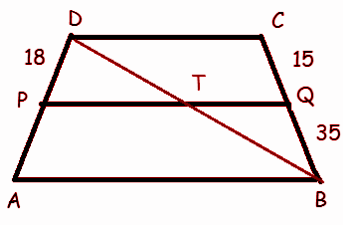In ΔDAB,

PD / PA = DT / TB —- (1)

In ΔDBC,

BT / TD = BQ / QC

On taking reciprocal on both sides,

TD / BT = QC / BQ —- (2)

(1) = (2)

PD / PA = QC / BQ

18 / PA = 15 / 35

PA = 18(35) / 15

PA = 42

Question 2: Rhombus PQRB is inscribed in ΔABC such that ∠B is one of its angles. P, Q and R lie on AB, AC and BC, respectively. If AB = 12 cm and BC = 6 cm, find the sides PQ, RB of the rhombus.

Solution: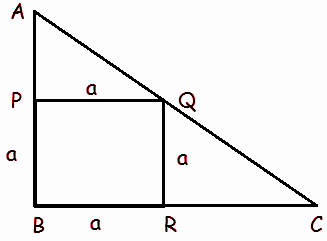In ΔABC, BC is parallel to PQ.

Let the side of the rhombus be a cm.

Given AB = 12 cm.

So AP = 12 – a

BC = 6 cm

AP / AB = PQ / BC

[12 – a] / 12 = a / 6

12a = 72 – 6a

18a = 72

a = 72 / 18

a = 4 cm

PQ = 4 cm and RB = 4 cm

Question 3: In ΔABC, AD is the bisector of ∠A meeting side BC at D, if AB = 10 cm, AC = 14 cm and BC = 6 cm, find BD and DC

Solution: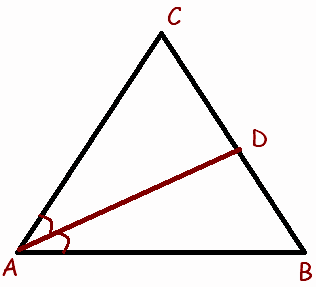AB / AC = DB / CD

Let DB = x, then CD = 6 – x

10 / 14 = x / (6-x)

5 / 7 = x / (6 – x)

5(6 – x) = 7x

30 – 5x = 7x

7x + 5x = 30

12x = 30

x = 30 / 12 = 5 / 2 = 2.5

BD = 2.5 cm and CD = 6 – 2.5 = 3.5 cm

Question 4: ABCD is a quadrilateral in which AB = AD, the bisector of ∠BAC and ∠CAD intersect the sides BC and CD at the points E and F respectively. Prove that EF is parallel to BD.

Solution: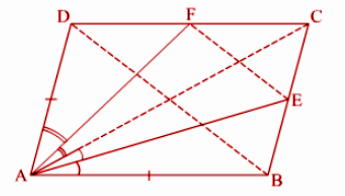In ΔABC, AE is the bisector of ∠BAC

AC / AB = CE / BE —- (1)

In ΔACD, AF is the bisector ∠CAD

AC / AD = CF / FD

AC / AB = CF / FD —- (2)

(1) = (2)

CE / BE = CF / FD

Hence EF is parallel to BD.

### Samacheer Kalvi 10th Maths Chapter 4: Geometry Book Exercise 4.3 Questions and Solutions

Question 1: A man goes 18 m due east and then 24 m due north. Find the distance of his current position from the starting point?

Solution: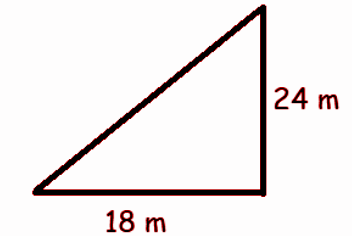The distance of his current position from the starting point

= √182 + 242

= √(324 + 576)

= √900

= 30 m

The required distance is 30 m.

Question 2: The hypotenuse of a right triangle is 6 m more than twice the shortest side. If the third side is 2 m less than the hypotenuse, find the sides of the triangle.

Solution:

Let x be the length of the shorter side.

Length of hypotenuse = 2x + 6

Length of third side = 2x + 6 – 2 = 2x + 4

(2x + 6)2 = x2 + (2x + 4)2

4x2 + 24x + 36 = x2 + 4x2 + 16x + 16

x2 + 16x – 24x + 16 – 36 = 0

x2 – 8x – 20 = 0

(x – 10) (x + 2) = 0

x = 10 and x = -2

Hence, the length of the shorter side is 10.

Hypotenuse = 2(10) + 6 = 26

Length of third side = 20 + 4 = 24

AC = √342 + 412

= √1156 + 1681

= √2837

= 53.26

Miles saved = (34 + 41) – 53.26

= 75 – 53.26

= 21.74 m

Question 3: The perpendicular PS on the base QR of a triangle PQR intersects QR at S, such that QS = 3 SR. Prove that 2PQ2 = 2PR2 + QR2.

Solution: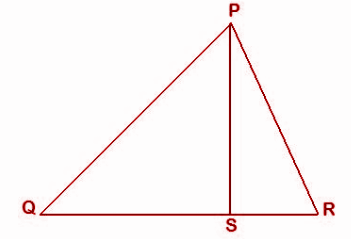QR = QS + RS

QR = 3RS + RS

QR = 4RS

RS = QR / 4

By applying Pythagorean theorem in ΔPQS,

PQ2 = PS2 + QS2

PS2 = PQ2 – QS2 —– (1)

By applying Pythagorean theorem in ΔPRS,

PR2 = PS2 + SR2

PS2 = PR2 – SR2 —– (2)

PQ2 – QS2 = PR2 – SR2

PQ2 – QS2 = PR2 – SR2

PQ2 = PR2 – SR2 + QS2

PQ2 = PR2 – SR2 + (QR – RS)2

PQ2 = PR2 – SR2 + QR2 + RS2 – 2 * QR * RS

PQ2 = PR2 + QR2 – 2 * QR * RS

PQ2 = PR2 + QR2 – 2 * QR x (QR / 4)

PQ2 = PR2 + QR2 – (QR2 / 2)

PQ2 = (2PR2 + 2QR2 – QR2) / 2

2PQ2 = 2PR2 + QR2

Question 4: In the adjacent figure, ABC is a right-angled triangle with right angle at B and points D, E trisect BC. Prove that 8AE2 = 3AC2 + 5AD2.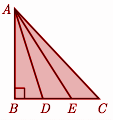Solution:

AE² = AB² + (2x)²

AE² = AB² + 4x²

In ΔABC,

AC² = AB² + BC²

AC² = AB + (3x)²

AC² = AB² + 9x²

Now,

3 AC² + 5AD² = 3(AB² + 9x²) + 5(AB² + x²)

= 8AB² + 32x²

= 8(AB² + 4x²)

= 8AE²8AE²

### Samacheer Kalvi 10th Maths Chapter 4: Geometry Book Exercise 4.4 Questions and Solutions

Question 1: The length of the tangent to a circle from a point P, which is 25 cm away from the centre is 24 cm. What is the radius of the circle?

Solution:

Let the centre of the circle be O.

OP = 25cm

Let the point of the tangent touching the circle be A.

AP = 24 cm

By Pythagoras theorem, the tangent to a circle touching the radius is perpendicular.

In ΔOAP,

(OP)² = (OA)² + (AP)²

25² = 24² + x²

x² = 625 – 576

x² = 49

x = 7cm

Question 2: A tangent ST to a circle touches it at B. AB is a chord such that ∠ABT = 65°. Find ∠AOB, where O is the centre of the circle.

Solution: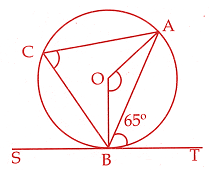Let C be the point on the circle on the opposite sides of the chord AB.

∠ABT = 65o

∠ACB = 65o [By alternate segment theorem]

∠AOB = 2 * 65o = 130o

∠AOB = 130o

Question 3: In two concentric circles, a chord of length 16 cm of larger circle becomes a tangent to the smaller circle whose radius is 6 cm. Find the radius of the larger circle

Solution: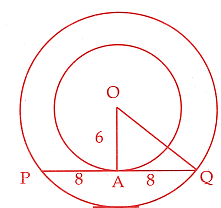Given that PQ = 16cm

AQ = 8cm

In ΔOAQ,

OQ2 = OA2 + AQ2

= 62 + 82

= 36 + 64

= 100

OQ = 10cm

The radius of the larger side is 10cm.

Question 4: Draw a tangent at any point R on the circle of radius 3.4 cm and centre at P?

Solution: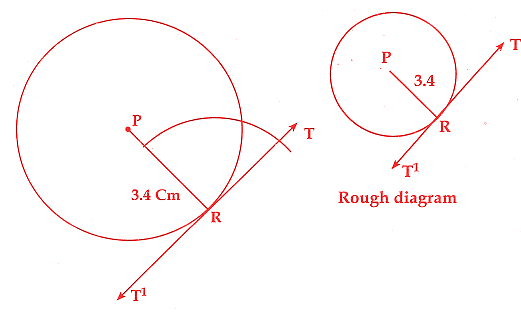Steps of construction:

1] Draw a circle of radius 3.4cm with centre P.

2] Mark a point R on the circle. Join PR.

3] Draw TT’ perpendicular to PR which passes through R.

4] TT’ is the tangent required.